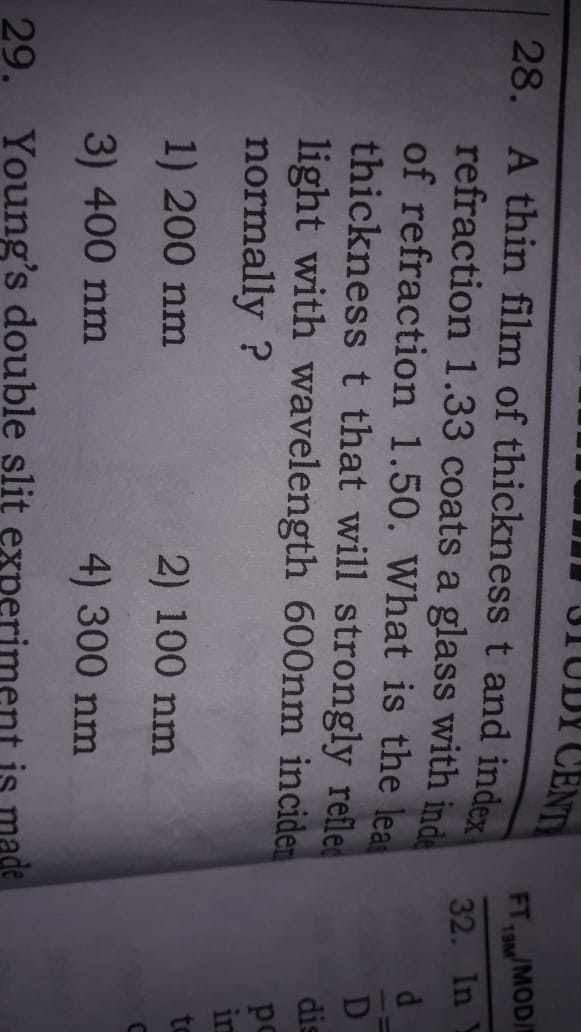# A thin film of thickness t and index of refraction 1.33 coats a glass with index of refraction 1.50.What is the least thickness t that will strongly reflect light with wavelength 600nm incident normally2 years ago
Expression of Least thickness t that will strongly reflect  light:

since, t = nλ/2μ

Here,

λ = Wavelength = 600 nm

μ = Refractive index of film = 1.33

For least thickness : n = 1

Hence,

t = λ / 2μ

= 600 / 2*1.33

= 600/ 2.66

= 225.56

≈ 225 nm

Thus, least thickness t that will strongly reflect  light with wavelength 600nm incident normally is 225 nm.

Thanks and regards
2 years ago

the condition is 2μtcosr = nλ

for normal incident r = 90

hence 2μt = nλ

so,  t = nλ/2μ

Here,

λ = Wavelength = 600 nm

μ = Refractive index of film = 1.33

For least thickness : n = 1

Hence,

t = λ / 2μ

= 600 / 2*1.33

= 600/ 2.66

= 225.56

≈ 225 nm

none of the options are correct. But we can take nearest answer whic is 200nm

2 years ago

the condition is 2μtcosr = nλ

for normal incident r = 0 deg

hence 2μt = nλ

so,  t = nλ/2μ

Here,

λ = Wavelength = 600 nm

μ = Refractive index of film = 1.33

For least thickness : n = 1

Hence,

t = λ / 2μ

= 600 / 2*1.33

= 600/ 2.66

= 225.56

≈ 225 nm

none of the options are correct. But we can take nearest answer whic is 200nm